ISSAC 96 - Hypergeometric Function Representations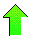Contents News View

 Results and Conclusion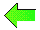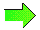The main accomplishment of our algorithm is the essential reproduction of 1504 formulas in 9 tables of representations of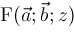listed in Integrals and Series, Volume 3: More Special Functions .  The total number of formulas in each of these tables is neatly summarized by the following table: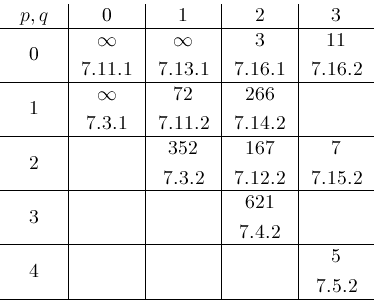The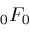,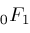, and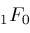entries are covered by general formulas.  The remaining 9 tables occupy most of the 186 pages of Chapter 7 material on hypergeometric functions.  Our algorithm can be used to extend these tables to values of parameters very far out from those given by Integrals and Series, Volume 3: More Special Functions .  The only limits on distance are the computer resources of time and memory.

The next table indicates the proportion of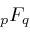formulas with parameters in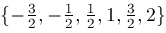that can be reduced by our algorithm.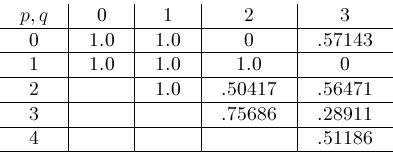This table means, for example, that our algorithm was able to compute 51.186 of the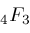's.  (Our algorithm does reduce other instances of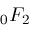and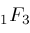, but none with the parameters mentioned here.)

In more recent work, our algorithm has been extended to compute representations for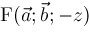, therefore making our algorithm encompass even more elementary and special functions.©2004-2021 Planet Quantum Kelly Roach